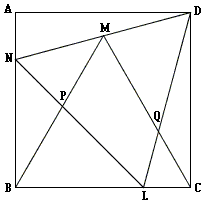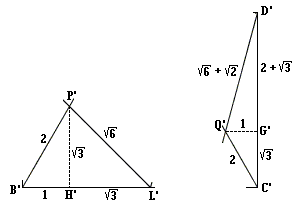# Equilateral Triangles and Incircles in a Square

Here is an elegant sangaku that requires both geometric and algebraic skills and some perseverance:Two equilateral triangles are inscribed into a square as shown in the diagram. Their side lines cut the square into a quadrilateral and a few triangles. Find a relationship between the radii of the two incircles shown in the diagram.

SolutionTwo equilateral triangles are inscribed into a square as shown in the diagram. Their side lines cut the square into a quadrilateral and a few triangles. Find a relationship between the radii of the two incircles shown in the diagram.

At first sight, the problem may appear surprising: do indeed equilateral triangles form such a configuration in a square? As a matter of fact they do so even in a more general setting. As a result, we may find all the angles in the diagram as shown below (in degrees):For the sake of convenience we label relevant points:and add four more: the projections of M, P, and Q on the sides of the square.Assume the side of the square equals 2: AB = BC = CD = AD = BM = CM = 2. Then in equilateral ΔBCM, the altitude MV = 3, the remainder MU = 2 - 3. From here (in ΔDUM) we can determine the values of trigonometric functions at 15° which will be useful later on. First

tan 15° = 2 - 3.

By the Pythagorean theorem,

 MD2 = UD2 + MU2 = 1 + (2 - √3)2 = 1 + 4 - 4√3 + 3 = 8 - 4√3 = 2·(4 - 2√3) = 2·(1 - √3)2

so that finally

MD = 2·(3 - 1) = 6 - 2.

Thus we can find sin 15° and cos 15°:

 sin 15° = UM / DM = (2 - √3) / (√6 - √2) = (√6 - √2) / 4 and, similarly, cos 15° = (√6 + √2) / 4.

Now continue. ΔDAN is a doubled replica of ΔDUM so that AN = 2(2 - 3). Therefore,

BN = BL = 2(3 - 1).

After these preliminaries we are in a position to tackle triangles BLP and CDQ. Because of a proliferation of radicals it is a convenience to begin with similar triangles B'L'P' and C'D'Q' in which B'H' = 1 and G'Q' = 1. The other lengths come out as follows:We apply the same standard formula as in another sangaku:

rp = 2S = ah,

where r, p, S, a, h are respectively the inradius, perimeter, area, a side and the altitude to the side in a triangle. In ΔB'L'P', the inradius is found to be

r' = 3·(1 + 3) / (3 + 3 + 6) = (1 + 3) / (1 + 2 + 3).

The inradius r1 of ΔBLP relates to r' as the ratio of the sides, e.g. BL/B'L' which is

BL/B'L' = 2(3 - 1) / (1 + 3) = 2(2 - 3),

from which

 (1) r1 = 2(√3 - 1) / (1 + √2 + √3).

Similarly, the inradius r2 of ΔCDQ can be found to be

 (2) r2 = 2 / (4 + 2√3 + √6 + √2).

With an additional effort, we conclude that (1) and (2) imply

r1 = 2r2.

And this is the relationship we were supposed to surmise from the diagram.

It goes without saying that any use of calculators (direct or implicit via dynamic geometry software) to solve this problem is rather inappropriate as the calculations would conceal rather than help discover patterns between various elements of the diagram. Check a remark on another page and the last chapter of an exceptional book by D. Niederman and D. Boyum.An anonymous visitor to the site has observed that the original diagram contains great many triangles, each with the incircle. The visitor drew attention to a pair of equal ones and conjectured that there are more such pairs of twin circles.The visitor has surmised that line PQ is perpendicular to LQ which shows that in the right ΔLPQ, angle at P is 30°, so that the hypotenuse LP is twice as long as the leg opposite angle P:

LP = 2·LQ.

Additionally triangles CQL and BLP are similar:In the similar triangles CQL and BLP a pair of corresponding sides (LP : LQ) is in the ratio 2 : 1 implying the same ratio for other linear elements. In particular, the inradii of the two triangles are in the ratio 2 : 1.

It follows that the incircles of triangles CQL and CDQ are equal. One then wonders whether another sangaku would not be more natural.However, simple comparison of the computed radii of various circles shows that besides the pair ADN and CDL of equal triangles, the diagram contains no twin circles in addition to the pair CQL and CDQ. Following is the collection of the radii of all the circles that appear in the double diagram above:

30.897424740513205
15.44871237025661
21.847778555217914
20.843374709207808
21.020708950586016
15.448712370256642
29.844620522684522
26.757954736802247
34.75479670426888
20.358888551841012
37.52222553867126
20.358888551840977
25.527816277595395
13.850936587751294
28.47257935337593

Most of the differences between the numbers in the list are sufficiently large to be explained by the accuracy (or the lack thereof) of calculations. Thus here is an example where the mathematical truth, albeit negative, can be established with the help of calculators.

### References

1. H. Fukagawa, D. Pedoe, Japanese Temple Geometry Problems, The Charles Babbage Research Center, Winnipeg, 1989

Write to:

Charles Babbage Research Center
P.O. Box 272, St. Norbert Postal Station
Winnipeg, MB

2. D. Niederman and D. Boyum, What The Numbers Say, Broadway Books, 2003## Sangaku• Equilateral Triangles on Sides of a Quadrilateral
• Euler Line Cuts Off Equilateral Triangle
• Four Incircles in Equilateral Triangle
• Problem in Equilateral Triangle
• Problem in Equilateral Triangle II
• Sum of Squares in Equilateral Triangle
• Triangle Classification
• Isoperimetric Property of Equilateral Triangles
• Maximum Area Property of Equilateral Triangles
• Angle Trisectors on Circumcircle
• Equilateral Triangles On Sides of a Parallelogram
• Pompeiu's Theorem
• Circle of Apollonius in Equilateral Triangle
•